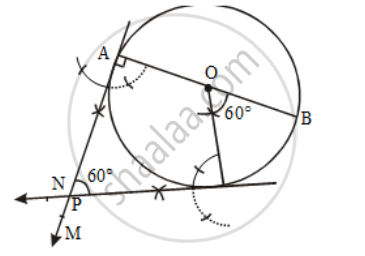Share

Books Shortlist

# Draw a circle of radius 3 cm. Draw a pair of tangents to this circle, which are inclined to each other at an angle of 60º. - CBSE Class 10 - Mathematics

ConceptConstruction of Tangents to a Circle

#### Question

Draw a circle of radius 3 cm. Draw a pair of tangents to this circle, which are inclined to each other at an angle of 60º.

#### Solution

Steps of constructionStep I: Draw a circle with O as centre and radius = 3 cm.

Step II: Draw any diameter AOB of this circle.

Step III: Construct ∠BOC = 60º such that radius OC meets the circle at C.

Step IV: Draw AM ⊥ AB and CN ⊥OC.

Let AM and CN intersect each other at P.

Then, PA and PC are the desired tangents to the given circle, inclined at an angle of 60º

Proof: ∠AOC = (180º – 60º) = 120º